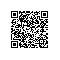# 九度题目1342：寻找最长合法括号序列II

(())()
(()

6
2

AC代码：

#include<stdio.h>
#include<string.h>
char s;
char stack;
int main()
{
int i,j,n,m,sum;
while(gets(s))
{
n=strlen(s);m=0;
sum=0;
for(i=0;i<n;i++)
{
stack[m++]=s[i];
if(m-2>=0)
{
if(stack[m-2]=='('&&stack[m-1]==')')
{
sum+=2;
m-=2;
}
}
}
printf("%d\n",sum);
}
return 0;
}使用钉钉扫一扫加入圈子
+ 订阅